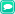## Posts Tagged “mathematics”

Well, loosely speaking, being continuous means that the function’s graph moves from one point to another along an unbroken path. If you were drawing the graph by hand, your pencil would never leave the paper as you moved from one point to another on the curve.

A technical definition used by mathematicians is:

A function f is continuous at a point c in its domain iff, given any epsilon greater than zero, there exists a delta greater than zero such that for each point in the domain lying within delta of c the functional value of that point lies within epsilon of the functional value at c.

Aren’t you glad you asked?No Comments »